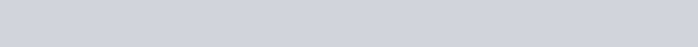# Python Variable

Filed Under: Python

In this tutorial we will know some basics about Python Variable. In our previous tutorial we discussed about Python print function.

## Python Variable

In your mathematics books, you probably heard about variables. Python Variables are use to store values.

When you declare a variable in python, some space regarding the variable is preserved in memory. Then you can access them. If you read our tutorial on Python data types, you should probably know about pythons data type.

### Python declare variable

In most common programming language like c, c++, java etc, you have to set the datatype of the variable when you declare the variable.

Python python is flexible about this. You can declare a variable and then the data-type of the variable depends on the data you’re storing into it. See the following example.

``````
# declare a variable
var = 'new variable'

print('The type of var is :',type(var))  # the type is str

var = 23.0
print('Now, the type of var is :', type(var))  # the type is float

``````

So you will see the output like this.### Multiple Variable Assignment

In our all these tutorials, you have never seen about these. Well, you saw about single variable value assignment.

But you can also assign values to multiple values altogether too. You have to keep the value in the rightmost side.

This idea is not used because we do not need to use this kind of assignment. But perhaps you project may need this idea and there is nothing wrong to learn new things. However, see the following code to understand multiple variable assignment.

``````
# assign multiple variable with the same value
var1 = var2 = var3 = 'init'

# print the value of each variable separately
print('Value of var1 :', var1)
print('Now, value of var1 :', var2)
print('Again, value of var1 :', var3)
``````

So, the output of the following code will be

``````
Value of var1 : init
Now, value of var1 : init
Again, value of var1 : init

``````

### Some notes about python variable

Some notes regarding creating a Python variable name is given below.

• Python variable names cannot be same as any python’s pre-defined keywords.
• The variable names should be written in camelCase

### Python print variable

We can use print() function to print the variable to console, as you have seen in above programs.

### Python variable scope

Python variable scope depends on where it’s declared in the program. We have explained python variable scope in more details in python namespace post.

That’s all for Python variable. For any query please use the comment box below.

close
Generic selectors
Exact matches only
Search in title
Search in content